# Lesson#52: Let’s check your lifeline with Excel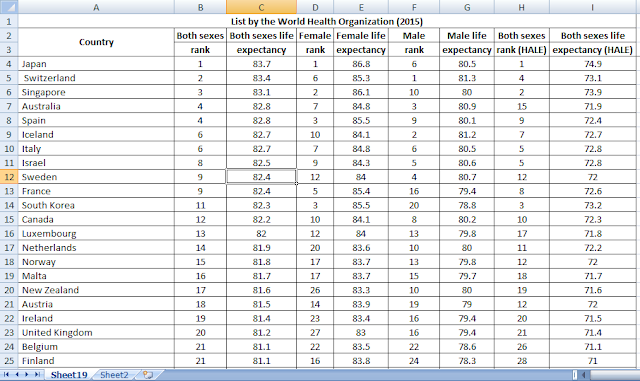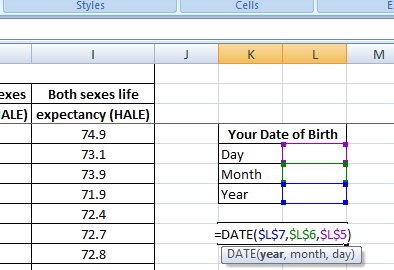Just a different exercise of excel. I have downloaded a list country wise average life expectancy by WHO. Lets check where are we in our lifeline by this sheet.

Lets take an input of date of birth from user. I have made different boxes for Year, Month and Day. From that it will make the date of birth by using this formula =IF(OR(\$B\$3=0,\$B\$4=0,\$B\$5=0),””,DATE(\$B\$5,\$B\$4,\$B\$3))
This formula won’t show any date of birth until any of the three box left blank.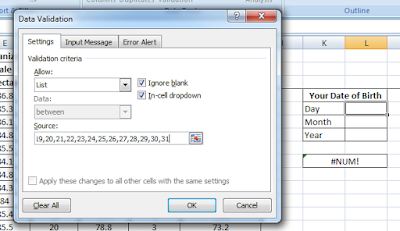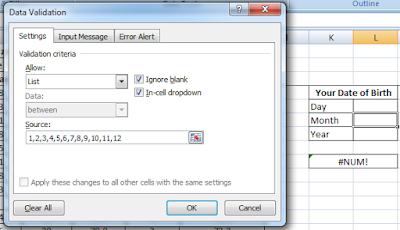Here input of day, month is to taken from the user will be selected from lists by using Data Validation just like I have shown in pictures.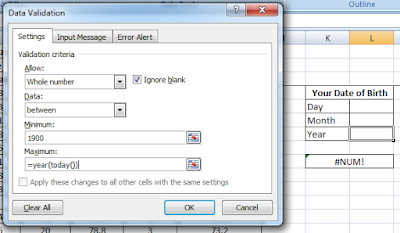For taking input of Year I have used Data Validation>Whole Number
I have given minimum value 1900 because excel doesn’t take value of Year before 1900 in date format. And the maximum value is =YEAR(TODAY()) the year of present date.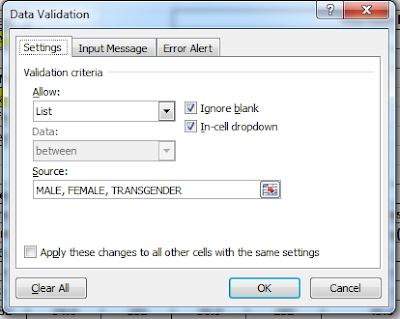For taking input of SEX here I have used a Data Validation>List which allows you to choose from a drop down list.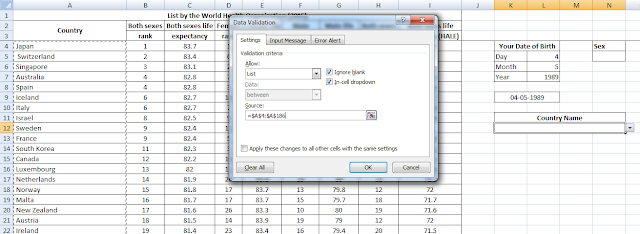For taking input of COUNTRY here I have used a Data Validation>List which allows you to choose from a drop down list.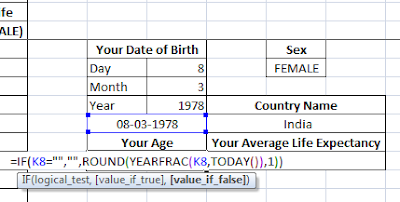Your Age is being calculated with this formula =IF(A6=””,””,ROUND(YEARFRAC(A6,TODAY()),1))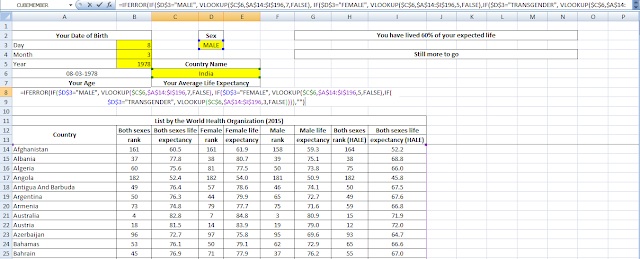=IFERROR(IF(\$D\$3=”MALE”, VLOOKUP(\$C\$6,\$A\$14:\$I\$196,7,FALSE), IF(\$D\$3=”FEMALE”, VLOOKUP(\$C\$6,\$A\$14:\$I\$196,5,FALSE),IF(\$D\$3=”TRANSGENDER”, VLOOKUP(\$C\$6,\$A\$14:\$I\$196,3,FALSE)))),””)

This formula lookup the value of Your Average Life Expectancy from the input of Sex and Country.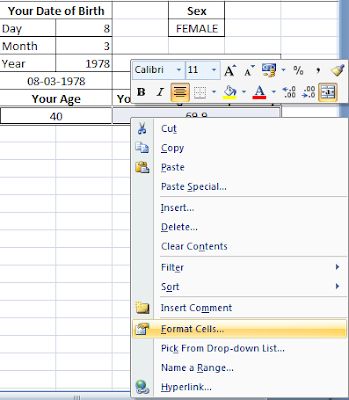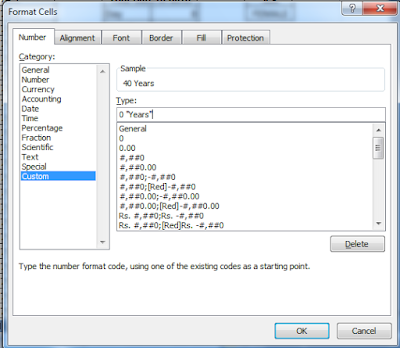Now I have selected both Your Age and Your Average Life Expectancy and right clicked on them. Selected Format Cells>Number>Custom and added suffix “Year”.

Then I have made two message box for showing the user.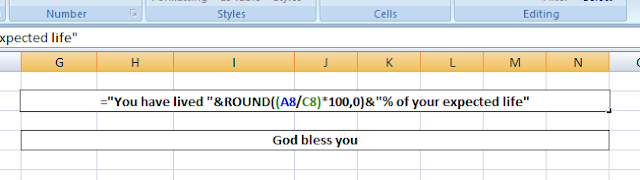This one deliver the message =IF(OR(\$B\$3=0,\$B\$4=0,\$B\$5=0,\$D\$3=0,\$C\$6=0),””,”You have lived “&ROUND((\$A\$8/\$C\$8)*100,0)&”% of your expected life”)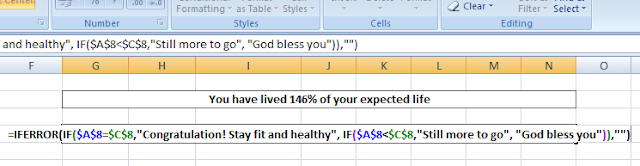Another one shows “Congratulation! Stay fit and healthy” if Your age = Your Average Life Expectancy,
“Still more to go” if Your age < Your Average Life Expectancy,
“God bless you” if Your age > Your Average Life Expectancy

by this formula =IF(OR(\$B\$3=0,\$B\$4=0,\$B\$5=0,\$D\$3=0,\$C\$6=0),””,IF(\$A\$8=\$C\$8,”Congratulation! Stay fit and healthy”, IF(\$A\$8<\$C\$8,”Still more to go”, “God bless you”)))